# $∞$ Infinity $∞$

## I. What is it that we call infinity ?

2. Hilbert’s Hotel

## II. How big is it ?

1. Counting to $∞$ : $\aleph_0$
2. Ordinals : $𝜔$
3. Inaccessible cardinals

Zeno of Elea : In a race, the quickest runner can never overtake the slowest.$⇑$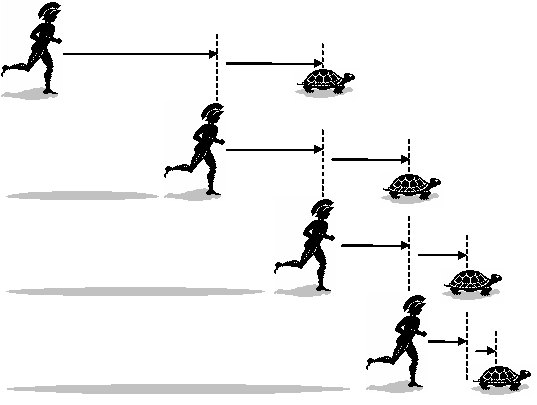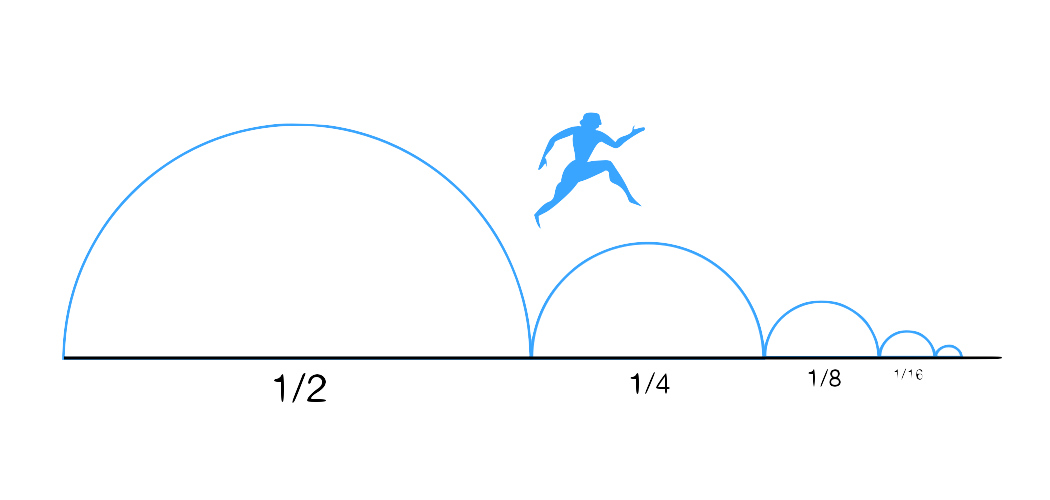Actually, modern math provide a solution :

$$\sum_{n>0} \frac{1}{2^n}$$ is convergent

# Hilbert's Hotel

• Fully occupied
• Infinitely many rooms

$⟶$ How many additional guests can be housed ?

1. For $n$ new guests : a shift of $n$
2. How about infintely many new guests ?# II. How big is infinity ?

## Cardinals : $\aleph_0$

Cardinal : the number of elements of an unordered set

$\downarrow$

If the set of all the integers ($ℕ$) exists, we call $\aleph_0$ its cardinal

NB : Zeno paradox$\aleph_0$ elements can be "written" within a finite space## Ordinals : $𝜔$

Ordinal : the first label you’ll have to use in order to append 1 element to an ordered set

NB :

• For a finite number of elements : ordinal ⟺ cardinal
• $\aleph_0 + 1 = \aleph_0$, but $𝜔 + 1 \neq 𝜔$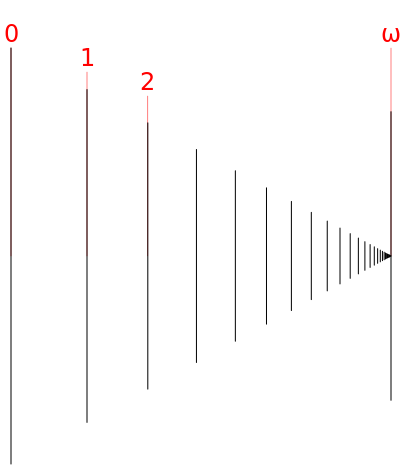### Axiom of replacement : to infinity and beyond !

Axiom of replacement : if you take an existing set and replace all elements with something else, you’re left with an other existing set.

We’re going to use it to the fullest !

$$𝜔^{2}$$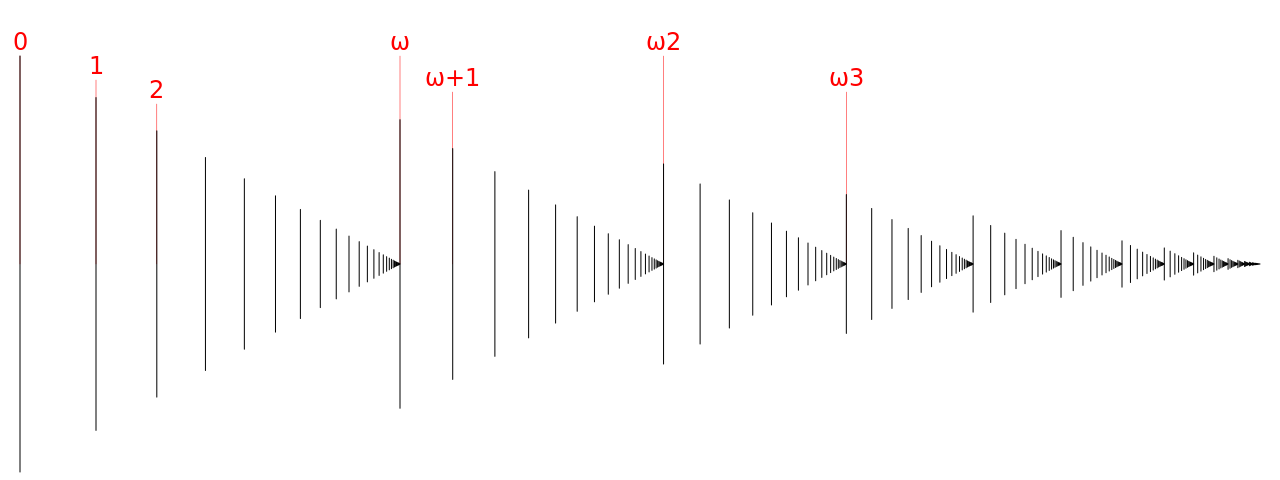$𝜔^3$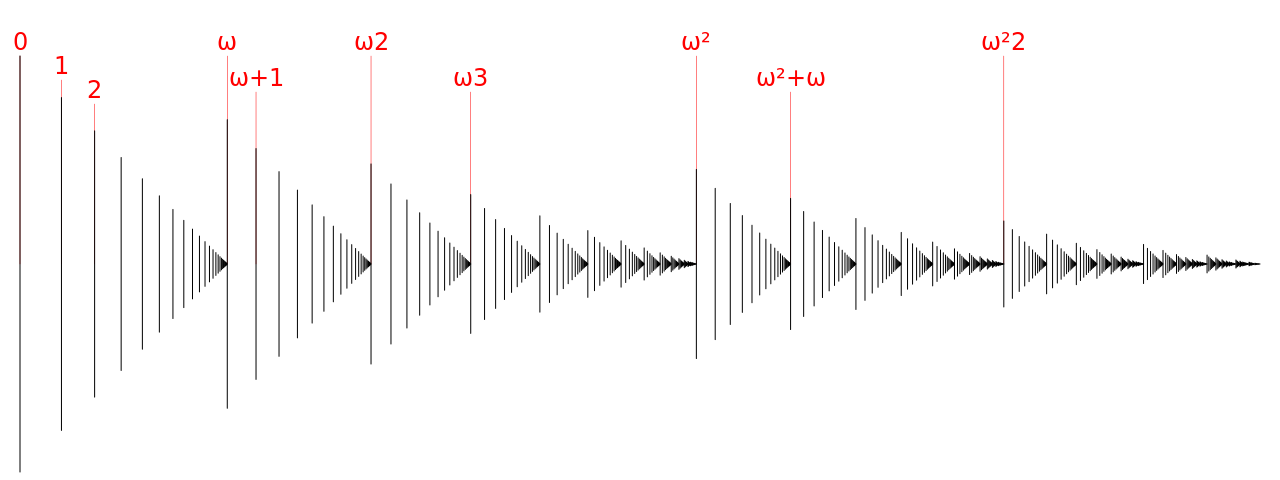$𝜔^4$$𝜔^𝜔$$𝜔^{5}$$𝜔^{𝜔^𝜔}$$𝜔^{𝜔^{𝜔^{\vdots^{𝜔}}}}$

up to

$𝜀_0$

# And all that is smaller than …

$\omega_1$

## corresponding to the cardinal …

$\aleph_1$

# Then …

$𝜔_2, 𝜔_3, \ldots, 𝜔_{𝜔}, \ldots, 𝜔_{𝜔^{𝜔^{𝜔^{\vdots^{𝜔}}}}}, 𝜔_{𝜀_0}, \ldots$

## and the corresponding cardinals

$\aleph_2, \aleph_3, \ldots$

# Power sets : becoming exponentially big

$|𝒫(E)| = 2^{|E|}$

+

Axiom of replacement

$\downarrow$

# But all of that has an order type … so : it remains smaller than …

Inaccessible cardinals